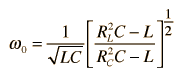# Parallel Resonance

The resonance of a parallel RLC circuit is a bit more involved than the series resonance. The resonant frequency can be defined in three different ways, which converge on the same expression as the series resonant frequency if the resistance of the circuit is small.Impedance definition Phase definition
Index

AC Circuits

 HyperPhysics***** Electricity and Magnetism R Nave
Go Back

# Resonance: Impedance Maximum

One of the ways to define resonance for a parallel RLC circuit is the frequency at which the impedance is maximum. The general case is rather complex, but the special case where the resistances of the inductor and capacitor are negligible can be handled readily by using the concept of admittance.

Index

AC Circuits

 HyperPhysics***** Electricity and Magnetism R Nave
Go Back

# Resonance: Phase Definition

Defining the parallel resonant frequency as the frequency at which the voltage and current are in phase, unity power factor, gives the following expression for the resonant frequency:The above resonant frequency expression is obtained by taking the impedance expressions for the parallel RLC circuit and setting the expression for Xeq equal to zero to force the phase to zero. After about a page of algebra, the above expression emerges. Note that for small values of the resistances, this approaches the series resonant frequency.

Index

AC Circuits

 HyperPhysics***** Electricity and Magnetism R Nave
Go Back